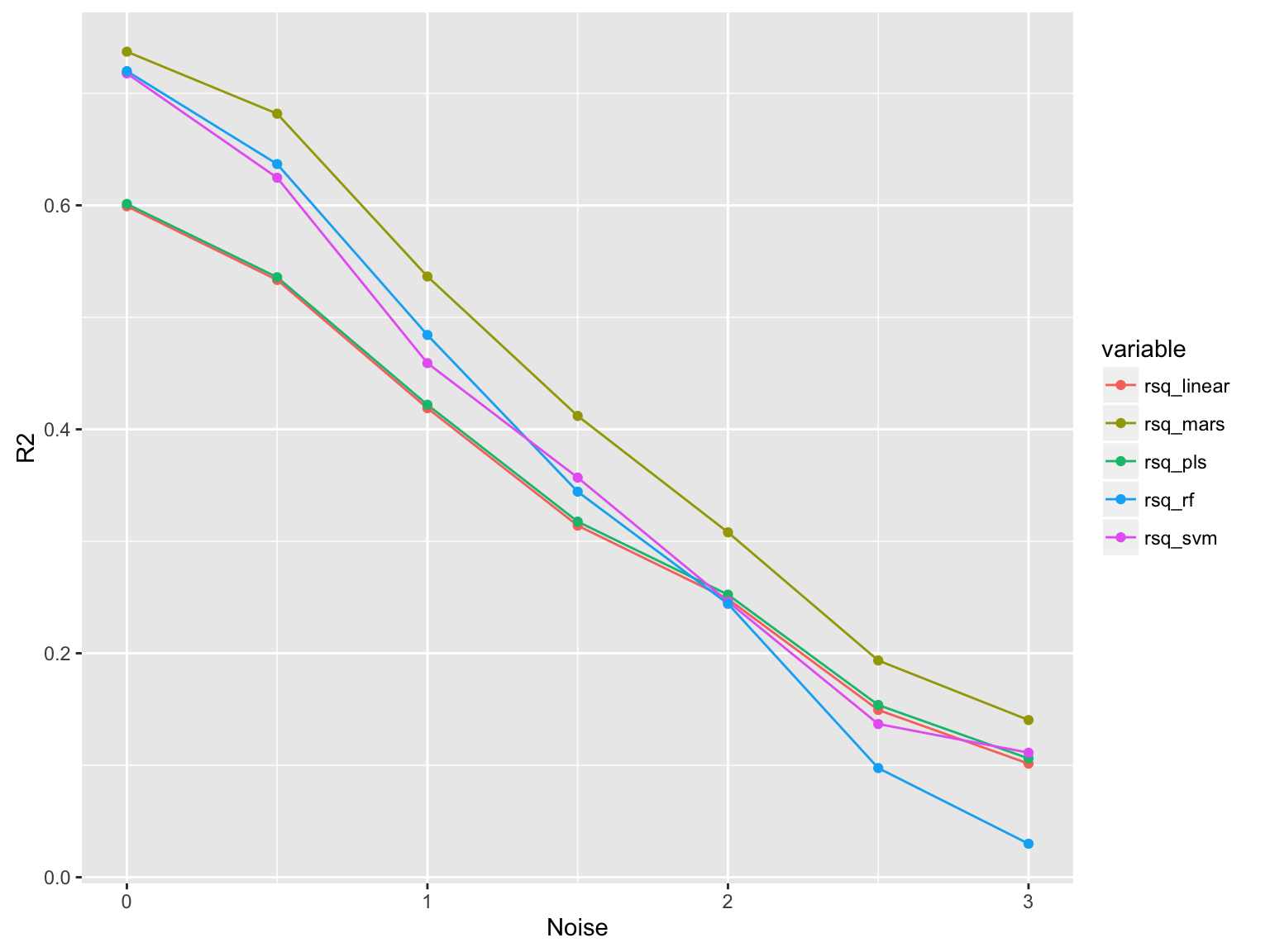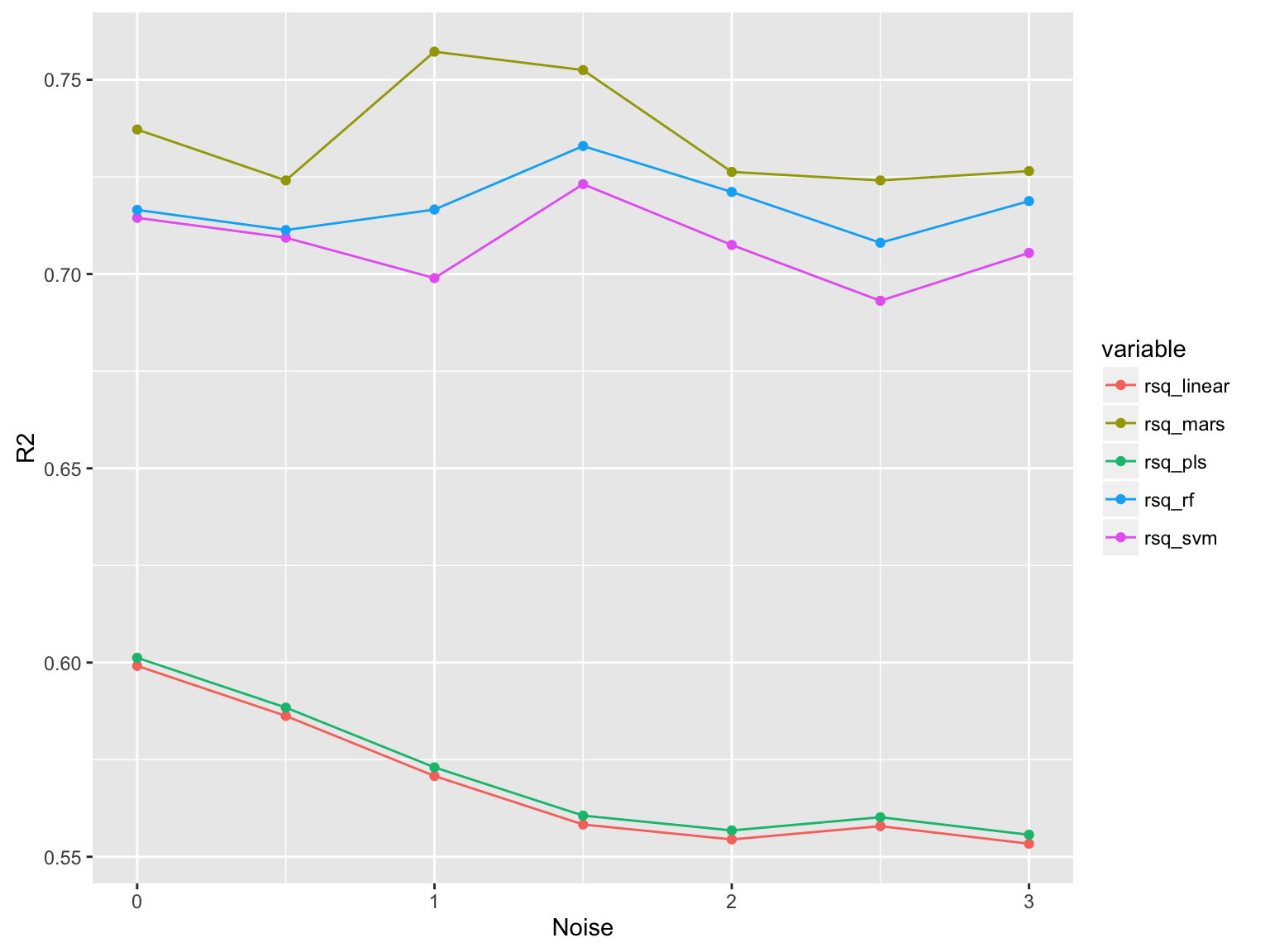2016-06-08

1. 什么是数据科学家
2. 数据预处理
3. 补充

# 介绍

• 幻灯片 & R代码：http://scientistcafe.com
• 数据整合 (Rcode1.R)
• psych包中的describe()函数
• apply()lapply()sapply()
• plyr包中的ddply()函数
• dplyr
• *reshape2
• *tidyr
• 建模技术 (Rcode2.R)
• 有监督 v.s 无监督
• 误差极其来源和影响
• *数据划分和再抽样
• *评估模型表现

# 服装消费者数据

1. 人口统计学变量
• 年龄（age）
• 性别（gender）
• 有房还是租房（house）
2. 消费者行为变量
• 2015年实体店购买该品牌服装花销（store_exp）
• 2015年在线购买该品牌服装花销（online_exp）
• 2015年实体店交易次数（store_trans）
• 2015年在线交易次数（online_trans）
3. 客户认知问卷调查

# 服装消费者数据

（Q1）：我喜欢买不同品牌的服装，比较它们
（Q2）：我喜欢买同一个品牌的服装
（Q3）：品牌的知名度对我来说非常重要
（Q4）：服装质量对我来说非常重要
（Q5）：服装风格我喜欢的风格
（Q6）：我喜欢在实体店购买
（Q7）：我喜欢在网上购买
（Q8）：价格对我来说很重要
（Q9）：我喜欢不同风格的衣服
（Q10）：我喜欢自己挑选服装，不需要周围人的建议

• 1（非常不同意）, 2（有点不同意）, 3（中立/不知道）, 4（有点同意）, 5（非常同意）

# 数据整合

• psych包中的describle()函数
• base包中的 apply()lapply()sapply()

1. 依次对某一对象的某一部分重复应用一个指定的函数
2. 返回对象不同
3. 有针对性的得到想要的输出结果
• plyr包中的ddply()函数

1. summarize
2. transform
3. subset

# 数据整合

• dplyr包: ddply()等函数的强化版，专门处理数据框（dataframe）对象，大幅提高了速度, 并且提供了更稳健的与其它数据库对象间的接口
1. 数据框显示
2. 数据截选（按行／列）
3. 数据总结
4. 生成新变量
5. 合并数据集

# 有监督 v.s 无监督学习

$\mathbf{y}=f(\mathbf{X})+\mathbf{\epsilon}$

1. 无监督建模：探索$$\mathbf{X}$$中的自变量之间的关系
2. 有监督建模：估计$$\mathbf{y}$$$$\mathbf{X}$$之间的关系 $$f(\cdot)$$

# 误差及其来源 - 系统误差和随机误差

• $$f$$的估计$$\hat{f}$$
• $$\mathbf{y}$$的预测 $$\hat{\mathbf{y}}=\hat{f}(\mathbf{X})$$
• 预测的误差分成两部分，系统误差和随机误差：

$E(\mathbf{y}-\hat{\mathbf{y}})^{2}=E[f(\mathbf{X})+\mathbf{\epsilon}-\hat{f}(\mathbf{X})]^{2}=\underset{\text{(1)}}{\underbrace{[f(\mathbf{X})-\hat{f}(\mathbf{X})]^{2}}}+\underset{\text{(2)}}{\underbrace{Var(\mathbf{\epsilon})}}$

（1）系统误差，能通过改进模型得到提高；
（2）随机误差，当前数据无法解释的部分，无法通过建立更复杂的模型来改进。

# 误差及其来源 - 应变量误差

• 反映在随机误差（$$\mathbf{\epsilon}$$）中
• 使得均方根误差（RMSE）和$$R^2$$有相应的上下限
• 假设我们希望利用消费记录变量建立关于消费者收入的预测模型

• 在应变量年收入（income）上添加不同程度的噪音（均方根误差的0到3倍）：

$RMSE \times (0.0, 0.5, 1.0, 1.5, 2.0, 2.5, 3.0)$

• 对以下模型的影响：一般线性回归，偏最小二乘回归，多元自适应回归样条，支持向量机（核函数是径向基函数），随机森林

# 误差及其来源 - 应变量误差# 误差及其来源 - 自变量误差

• 自变量观测中的随机性产生的影响取决于如下几个因素：

1. 随机性的强度
2. 相应因变量在模型中的重要性
3. 使用模型的类别
• 在自变量online_exp和上添加如下不同程度的噪音（该变量观测标准差的0到3倍）

$\sigma_{0} \times (0.0, 0.5, 1.0, 1.5, 2.0, 2.5, 3.0)$

• 检查噪音强度对复杂度不同的模型拟合$$R^2$$的影响

• 一般线性回归，偏最小二乘回归，多元自适应回归样条，支持向量机（核函数是径向基函数），随机森林

# 误差及其来源 - 自变量误差# 数据整合和辅助建模技术（2）

• 数据整形
• reshape2
• tidyr
• 建模技术
• 数据划分
• 按照结果变量划分数据
• 按照自变量划分
• 按时间序列划分
• 数据重抽样
• k折交叉验证
• 重复训练/测试集划分
• Bootstrap
• 评估模型表现
• 回归模型
• 分类模型

# 参考文献（数据预处理）

• Ton de Waal, Sander Scholtus, Jeroen Pannekoek. 2011. Handbook of Statistical Data Editing and Imputation. John Wiley & Sons.
• Jolliffe, I.T. 2002. Principla Component Analysis. 2nd ed. New York: Springer.
• Box G, Cox D. 1964. “An Analysis of Transformations.” Journal of the Royal Statistical Society Series B (Methodological): 211–52.
• Iglewicz, Boris, and David Hoaglin. 1993. “How to Detect and Handle Outliers.” The ASQC Basic References in Quality Control: Statistical Techniques 16.
• Serneels S, Espen PV, Nolf ED. 2006. “Spatial Sign Pre-Processing: A Simple Way to Impart Moderate Robustness to Multivariate Estimators.” Journal of Chemical Information and Modeling 46 (3): 1402–9.
• Kjell Johnston, Max Kuhn &. 2013. Applied Predictive Modeling. Springer.